# NCERT Solutions for Chapter 6 Be My Multiple, I'll Be Your Factor Class 5 MathsChapter Name Be My Multiple, I'll Be Your Factor NCERT Solutions Class CBSE Class 5 Textbook Name Maths Related Readings NCERT Solutions for Class 5NCERT Solutions for Class 5 Maths Summary of Be My Multiple, I'll Be Your Factor

Page No 87:

Question 1: The hungry cat is trying to catch Kunjan the mouse. Kunjan is now on the 14th step and it can jump 2 steps at a time. The cat is on the third step. She can jump 3 steps at a time. If the mouse reaches 28 it can hide in the hole. Find out whether the mouse can get a way safely!

(a) The steps on which the mouse jumps ________________

(b) The steps on which the cat jumps. ________________

(c) The steps on which both the cat and the mouse jump

(d) Can the mouse get away?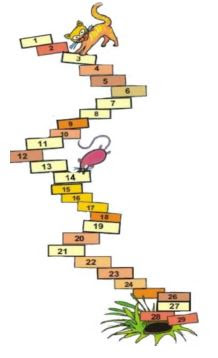Find out If the cat starts from the 5th step and jumps five steps at a time and the mouse starts from the 8th step and jumps four steps at a time, can the mouse get away?

(a) The steps on which the mouse jumps 16, 18, 20, 22, 24, 26, 28

(b) The steps on which the cat jumps 6, 9, 12, 15, 18, 21, 24, 27

(c) The steps on which both the cat and the mouse jump 18 and 24

(d) Yes, the mouse can get away.

If the cat starts from 5th step, and jumps 5 steps at a time, the cat will reach 10, 15, 20 and 25 steps. If the mouse starts from 8th step, and jumps 4 steps at a time, the mouse will reach 12, 16, 20 and 24 steps.

So, the cat will easily be able to catch the mouse at step 20. This time, the mouse cannot get away.

Page No 88:

Question 1: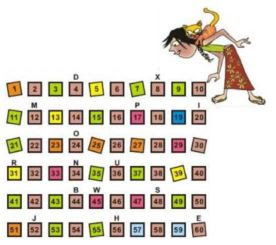Mark with a red dot all the numbers which can be divided by 2.

Mark a yellow dot on the numbers which can be divided by 3 and a blue

dot on the numbers which can be divided by 4.

Which are the boxes which have dots of all three colours?

What are the letters on top of those boxes?

Write those letters below in order.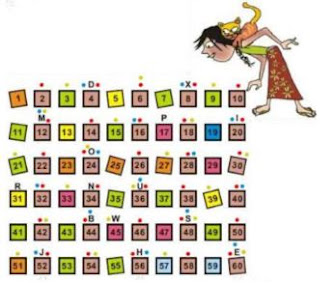Boxes 12, 24, 36, 48 and 60 have dots of all the 3 colours. Letters on top

of those boxes are M, O, U, S and E.

Letters in order will be E, M, O, S and U.

Page No 89:

Question 1: To play this game, everyone stands in a circle. One player calls out 'one'. The next player says 'two' and so on. A player who has to call out 3 or a number which can be divided by 3 has to say 'Meow' instead of the number. One who forgets to say 'Meow' is out of the game. The last player left is the winner. Which numbers did you replace with 'Meow'? 3, 6, 9.......................

The numbers replaced by word "Meow" are 3, 6, 9, 12, 15, 18, 21, 24, 27, 30 and so on.

Question 2: We say these numbers are the multiples of 3. Play the game by changing the number to 4. Now, which numbers did you replace with 'Meow"? These numbers are the multiples of 4.Write any ten multiples of 5.

Numbers that get replaced with "Meow" are 4, 8, 12, 16, 20, 24, 28, 32, 36, 40 and so on.

Ten multiples of 5 are:

 5, 10, 15, 20, 25, 30, 35, 40, 45 and 50.

Page No 90:

Question 1: Throw two dice together. What are the numbers that turn up on the faces of the dice? Make a two-digit number using them. If it is a multiple of any of the numbers written next to the circles, you can write it in that circle.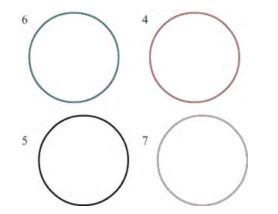Disclaimer: The answer may vary from student to student, based on his/her observation. It is highly recommended that the students prepare the answer on their own.

Page No 91:

Question 1: Think of a number. If it is a multiple of 3 write it in the red circle. If it is a multiple of 5 write it in the blue circle.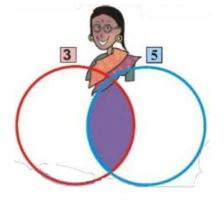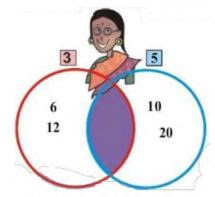Disclaimer: The answer may vary from student to student, based on his/her observation. It is highly recommended that the students prepare the answer on their own. The answer provided here is for reference only.

Question 2: If you write the multiples common to 3 and 5 in the purple part, then will they still be in both the red and the blue circles?

• Which is the smallest among these common multiples? __________

Repeat the game using the numbers 2 and 7.

• Write the common multiples of 2 and 7.

• Smallest among the common multiples will be 15.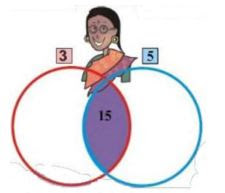Common multiples of 2 and 7 are 14, 28, 42, 56 and so on.

Disclaimer: The answer of the first part of the question may vary from student to student, based on his/her observation. It is highly recommended that the students prepare the answer on their own. The answer provided here is for reference only.

Page No 92:

Question 1: Putting the multiples of 4, 6 and 5 in the circles.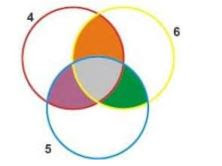• What common multiples of 5 and 6 did you write in the green part?
• What common multiples of 4 and 6 are written in the orange part?
• In which coloured part did you write the common multiples of 4, 6 and 5?
• What is the smallest common multiple of 4, 6 and 5? ________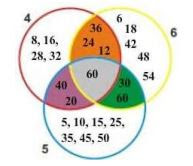• Common multiples of 5 and 6 are 30 and 60, which is written in green part.
• Common multiples of 4 and 6 are 12, 24 and 36, which is written in orange part.
• Common multiples of 4, 5 and 6 are written in grey part.
• Smallest common multiple of 4, 5 and 6 is 60.

Disclaimer: Students can write many common multiples of 5 and 6 or 4 and 6. The answers provided here are for reference only.

Question 2: Sunita took some tamarind (imli) seeds. She made groups of five with them, and found that one seed was left over. She tried making groups of six and groups of four. Each time one seed was left over. What is the smallest number of seeds that Sunita had?

We know, that if we make groups of 4, 5 and 6, each time we will be left with 1 seed. So, we calculate the LCM of 4, 5 and 6 and add 1 to it. Thus, the LCM of (4, 5, 6) = 60 Adding 1 to it, we get 61.

So, the smallest possible number of seeds that Sunita had is 61.

Page No 93:

Question 1: Ammini is arranging 12 tamarind seeds in the form of different rectangles. Try to make more rectangles like this using 12 tamarind seeds. How many different rectangles can you make?

Different rectangles that can be made from 12 tamarind seeds are as follows: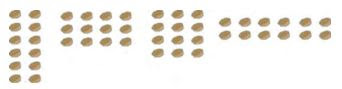Thus, we can make five different rectangles.

Question 2: If there are 15 tamarind seeds how many rectangles can you make?

We can make two rectangles from 15 tamarind seeds in the following way: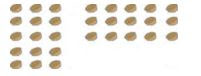Thus, we can make two rectangles.

Question 3: Colouring the Grid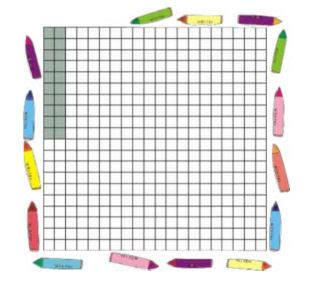In the grid here, a rectangle made of 20 boxes is drawn. The width of this rectangle is 2 boxes.

• What is its length?
• Colour a rectangle made of 20 boxes in some other way.

• Length of the rectangle is 10 boxes.
• Following are the different ways of making a rectangle with 20 boxes: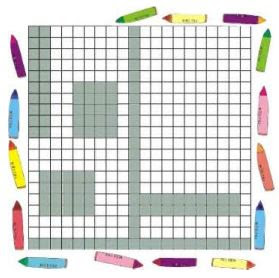Disclaimer: We can colour the rectangle of 20 boxes in many other ways. The answer provided here is for reference only.

Page No 94:

Question 1:

• What is the length and width of the rectangle you coloured?
• In how many ways can you colour a rectangle of 20 boxes? Colour them all in the grid, and write the length and width of each rectangle you have coloured.

• In the first case, the length and breadth of coloured rectangle is 5 and 4 boxes. In the second case, the length and breadth of coloured rectangle is 10 and 2 boxes.

Question 2: There are 18 bangles on the road. Meena is trying to group them. She can put them in groups of 2, 3, 6, 9 and 18

• without any bangle being left.
• How many groups will she have if she makes groups of 1 bangle each? ______ Now complete the table, for different numbers of bangles. For each number see what different groups can be made.
 Number of bangles Different groups we can make 18 1,2,3,6,9,18 24 1,2,…………… 5 9 7 2 10 1 20 13 21

• If she makes groups of 1 bangle each, she will get 18 groups.
 Number of bangles Different groups we can make 18 1,2,3,6,9,18 24 1,2,3,4,6,8,12,24 5 1,5 9 1,3,9 7 1,7 2 1,2 10 1,2,5,10 1 1 20 1,2,4,5,10,20 13 1,13 21 1,3,7,21

Page No 95:

Question 1: Complete the multiplication chart given here.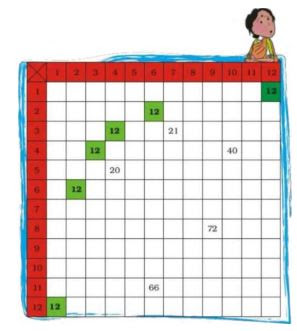Look at the green boxes in the chart. These show how we can get 12 by multiplying different numbers.

 12 4 × 3    6 × 2    1 × 12

12 = 4 x 3, so 12 is a multiple of both 4 and 3. 12 is also a multiple of 6 and 2, as well as 12 and 1. We say 1, 2, 3, 4, 6, 12 are factors of 12.

• What are the factors of 10? ______

 10 5 × 2 _ _ _ _

Can you do this from the chart?

• What are the factors of 36? ______
• Find out all the factors of 36 from the multiplication chart.
• What is the biggest number for which you can find the factors form this chart?
• What can you do for numbers bigger than that?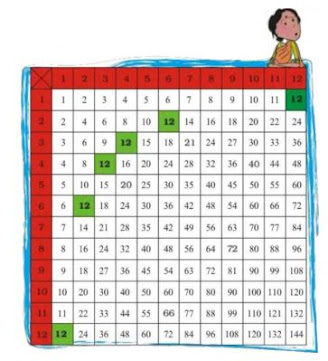• Factors of 10 are 1, 2, 5 and 10.

 10 5 × 2 1 × 10

• Factors of 36 are 1, 2, 3, 4, 6, 9, 12, 18 and 36.

 36 2 × 18 3  × 12 1  × 36 4  × 9 6  × 6

• 144 is the largest number for which we can find out factors from this chart.

•  For numbers larger than this, we will extend our multiplication chart, both row wise and column wise, and then complete the chart.

Page No 96:

Question 1: Write the factors of 25 in the red circle and the factors of 35 in the blue circle.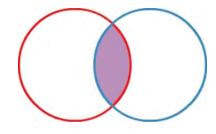Which are the factors you have written in the common part (purple) of both circles? These are common factors of 25 and 35.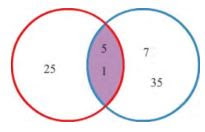1 and 5 are the common factors of 25 and 35. Thus, it is written in the common part of both the circles.

Question 2: Now write the factors of 40 in the red circle and 60 in the blue circle.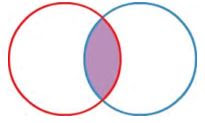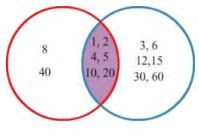Page No 97:

Question 1: Look at the factor tree. Now can you make another tree like this?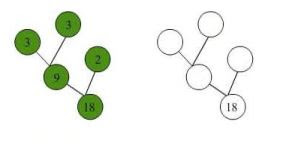In how many ways can you draw a factor tree for 24? Draw three of them below.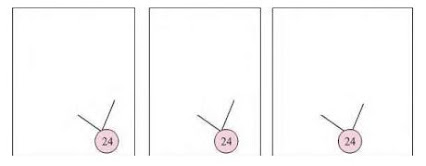••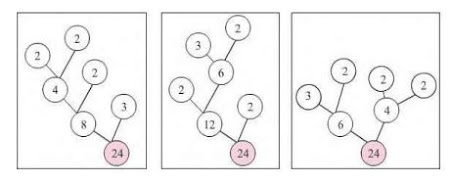Question 2: There is a garden in Anu's house. In the middle of the garden there is a path. They decided to tile the path using tiles of length 2 feet, 3 feet and 5 feet.

The mason tiled the first row with 2 feet tiles, the second row with 3 feet tiles and the third row with 5 feet tiles. The mason has not cut any of the tiles. Then what is the shortest length of the path?

Multiples of 2 = 2, 4, 6, 8, 10, 12, 14, 16, 18, 20, 22, 24, 26, 28, 30. Multiples of 3 = 3, 6, 9, 12, 15, 18, 21, 24, 27, 30. Multiples of 5 = 5, 10, 15, 20, 25, 30

Smallest common multiple is 30. So, the shortest length of the path is 30 m.

Page No 98:

Question 1: Manoj has made a new house. He wants to lay tiles on the floor. The size of the room is 9 feet x 12 feet. In the market, there are three kinds of square tiles: 1 foot × 1 foot, 2 feet × 2 feet and 3 feet × 3 feet. Which size of tile should he buy for his room, so that he can lay it without cutting?

Size of the room = 9 feet x 12 feet. And there are 3 kinds of square tiles, 1 foot × 1 foot, 2 feet × 2 feet and 3 feet × 3 feet. Now, size of tile that he should choose to lay it without cutting should be a factor of 9 and 12 both. As we know, I is a factor of all the numbers, so he can buy tiles of measure 1 foot × 1 foot. Now, 2 is not a factor of 9, so tiles of measure 2 foot × 2 foot cannot be laid without cutting. Also, we know 3 is a factor of 9 and 12 both, so he can also choose the tiles of measure 3 feet × 3 feet. Thus, he can buy tiles with measure 1 foot x 1 foot or 3 feet x 3 feet for his room.

Question 2: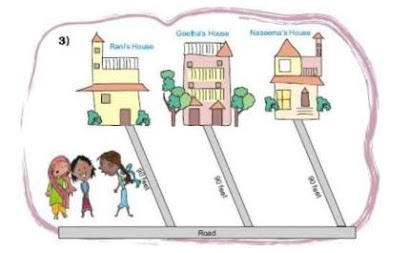Rani, Geetha and Naseema live near each other. The distance from their houses to the road is 90 feet. They decided to tile the path to the road. They all bought tiles of different designs and length. Rani bought the shortest tile, Geetha bought the middle sized one and Naseema bought the longest one. If they could tile the path without cutting any of the tiles,
what is the size of the tiles each has bought? Suggest 3 different solutions. Explain how you get this answer.

Length of the path = 90 feet Now, they have to choose a size of tile that can be tiled on the path without cutting. So, size of tile should be a factor of 90.

Factors of 90 are 1, 2, 3, 5, 6, 9, 10, 15, 18, 30, 45 and 90. Rani chooses the smallest size tile, Geetha takes the middle sized and Naseema the largest.

So, we can choose the tiles in the following way:

(a) Rani can take tiles of size 1 foot x 1 foot,

Geetha can choose tiles of size 2 feet x 2 feet,

Naseema can buy tiles of size 3 feet × 3 feet.

(b)Rani can take tiles of size 2 feet × 2 feet,

Geetha can choose tiles of size 3 feet x 3 feet,

Naseema can buy tiles of size 5 feet × 5 feet.

(c) Rani can take tiles of size 3 feet × 3 feet,

Geetha can choose tiles of size 5 feet × 5 feet,

Naseema can buy tiles of size 6 feet × 6 feet.